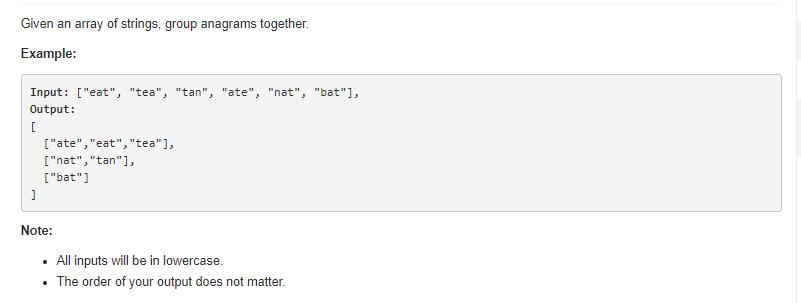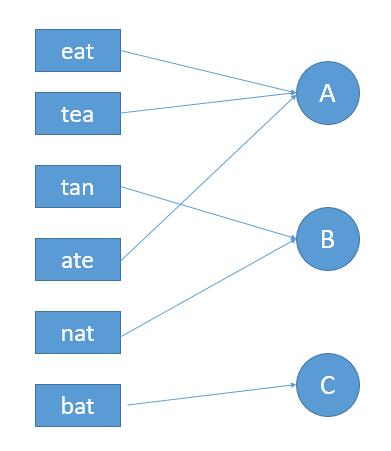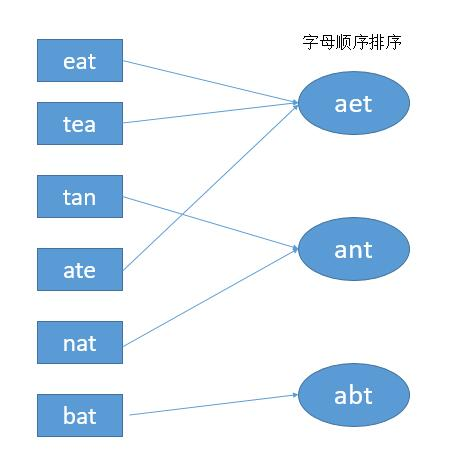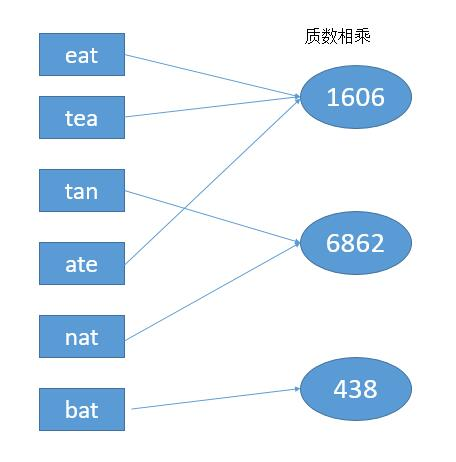# 题目描述（中等难度）# 解法一

public List<List<String>> groupAnagrams(String[] strs) {
List<List<String>> ans = new ArrayList<>();
boolean[] used = new boolean[strs.length];
for (int i = 0; i < strs.length; i++) {
List<String> temp = null;
if (!used[i]) {
temp = new ArrayList<String>();
//两两比较判断字符串是否符合
for (int j = i + 1; j < strs.length; j++) {
if (equals(strs[i], strs[j])) {
used[j] = true;
}
}
}
if (temp != null) {

}
}
return ans;

}

private boolean equals(String string1, String string2) {
Map<Character, Integer> hash = new HashMap<>();
//记录第一个字符串每个字符出现的次数，进行累加
for (int i = 0; i < string1.length(); i++) {
if (hash.containsKey(string1.charAt(i))) {
hash.put(string1.charAt(i), hash.get(string1.charAt(i)) + 1);
} else {
hash.put(string1.charAt(i), 1);
}
}
//记录第一个字符串每个字符出现的次数，将之前的每次减 1
for (int i = 0; i < string2.length(); i++) {
if (hash.containsKey(string2.charAt(i))) {
hash.put(string2.charAt(i), hash.get(string2.charAt(i)) - 1);
} else {
return false;
}
}
//判断每个字符的次数是不是 0 ，不是的话直接返回 false
Set<Character> set = hash.keySet();
for (char c : set) {
if (hash.get(c) != 0) {
return false;
}
}
return true;
}# 解法二public List<List<String>> groupAnagrams(String[] strs) {
HashMap<String, List<String>> hash = new HashMap<>();
for (int i = 0; i < strs.length; i++) {
char[] s_arr = strs[i].toCharArray();
//排序
Arrays.sort(s_arr);
//映射到 key
String key = String.valueOf(s_arr);
//添加到对应的类中
if (hash.containsKey(key)) {
} else {
List<String> temp = new ArrayList<String>();
hash.put(key, temp);
}

}
return new ArrayList<List<String>>(hash.values());
}


# 解法三public List<List<String>> groupAnagrams(String[] strs) {
HashMap<Integer, List<String>> hash = new HashMap<>();
//每个字母对应一个质数
int[] prime = { 2, 3, 5, 7, 11, 13, 17, 19, 23, 29, 31, 41, 43, 47, 53, 59, 61, 67, 71, 73, 79, 83, 89, 97, 101, 103 };
for (int i = 0; i < strs.length; i++) {
int key = 1;
//累乘得到 key
for (int j = 0; j < strs[i].length(); j++) {
key *= prime[strs[i].charAt(j) - 'a'];
}
if (hash.containsKey(key)) {
} else {
List<String> temp = new ArrayList<String>();
hash.put(key, temp);
}

}
return new ArrayList<List<String>>(hash.values());
}


# 解法四

"cdc" 就映射到了 "0#0#2#1#0"。

"dcc" 就映射到了 "0#0#2#1#0"。

public List<List<String>> groupAnagrams(String[] strs) {
HashMap<String, List<String>> hash = new HashMap<>();
for (int i = 0; i < strs.length; i++) {
int[] num = new int;
//记录每个字符的次数
for (int j = 0; j < strs[i].length(); j++) {
num[strs[i].charAt(j) - 'a']++;
}
//转成 0#2#2# 类似的形式
String key = "";
for (int j = 0; j < num.length; j++) {
key = key + num[j] + '#';
}
if (hash.containsKey(key)) {
} else {
List<String> temp = new ArrayList<String>();
hash.put(key, temp);
}

}
return new ArrayList<List<String>>(hash.values());
}